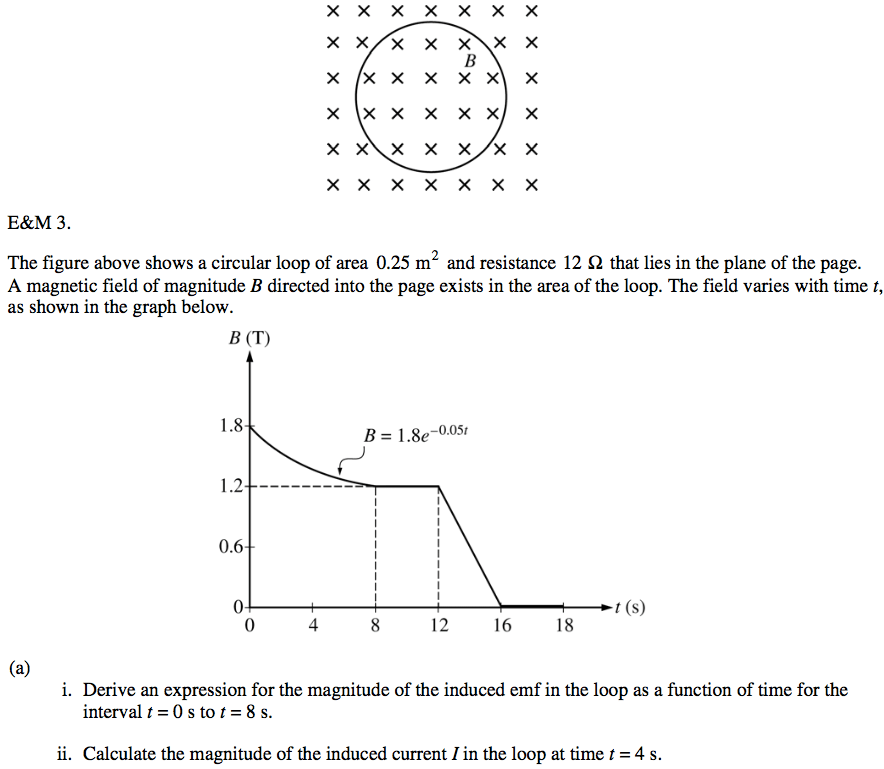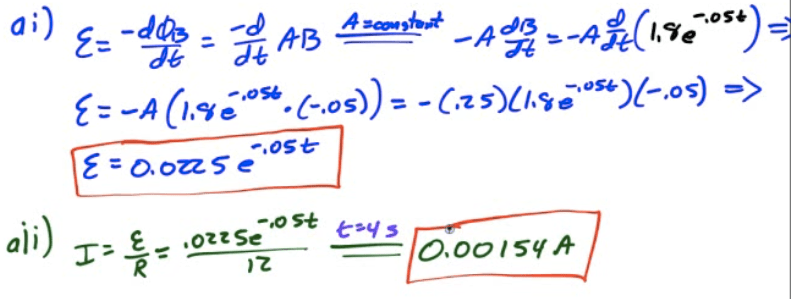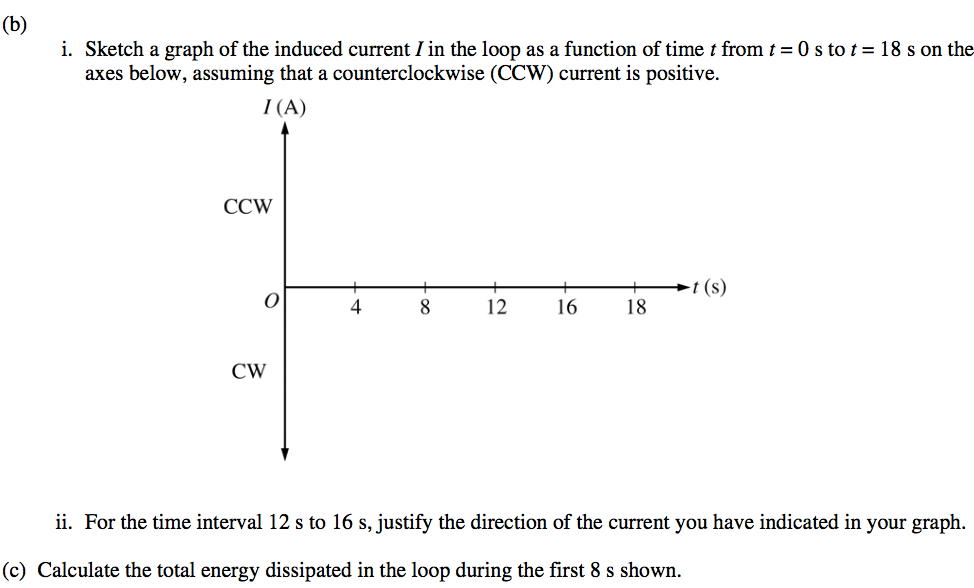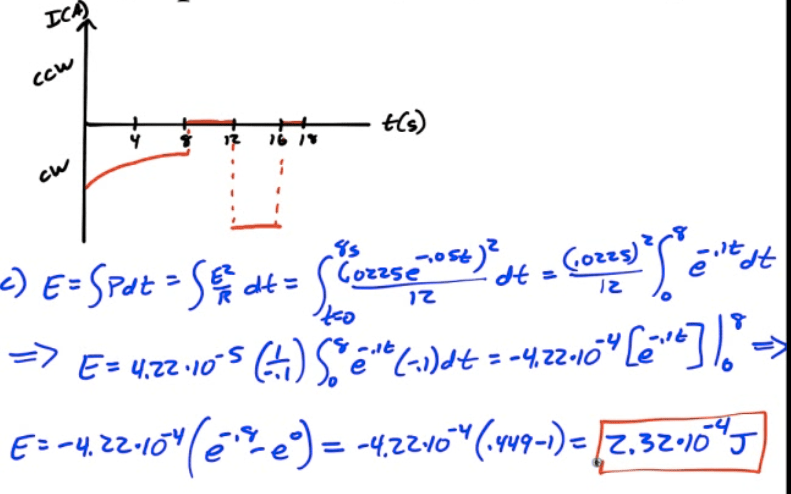• The induced emf due to a changing magnetic field is equal in magnitude to the rate of change of the magnetic flux through a surface bounded by the circuit

• The direction of the induced current is given by Lenz's Law

•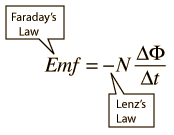# Lenz's Law

• The current induced by a changing magnetic flux creates a magnetic field opposing the change in flux# Maxwell's Equations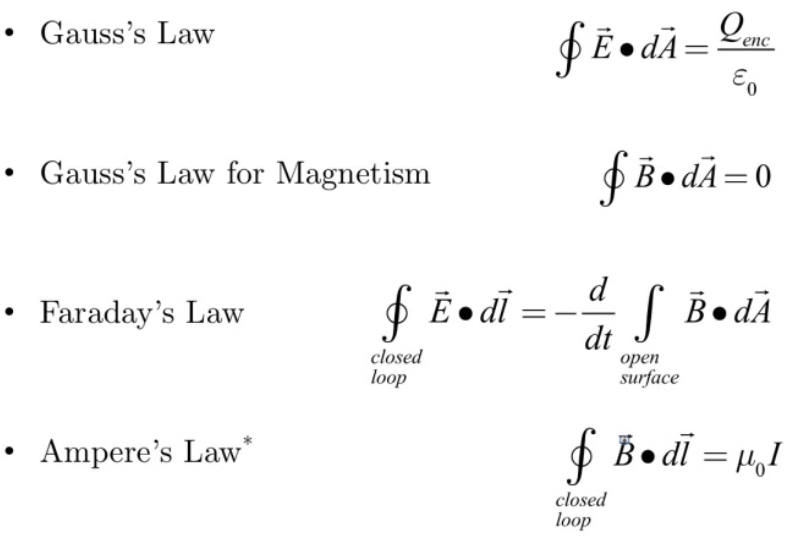# Example 1: Induced Current in a Loop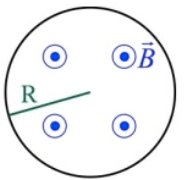•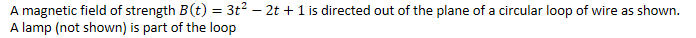1. Find the generated emf as a function of time

•2. Determine the current through the 100-ohm lamp as a function of time

•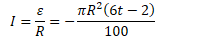3. What is the direction of the current through the loop at time t=5s?

•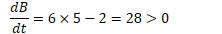• Clockwise, due to Lenz's Law

# Example 2: Rod on Rails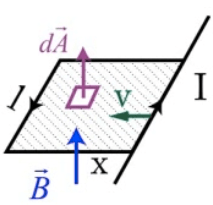• Consider a circuit in which a current-carrying rod on rails is moved to the left with constant velocity v. If the circuit is perpendicular to a constant magnetic field, determine the induced emf in the circuit

•# 2012 Free Response Question 3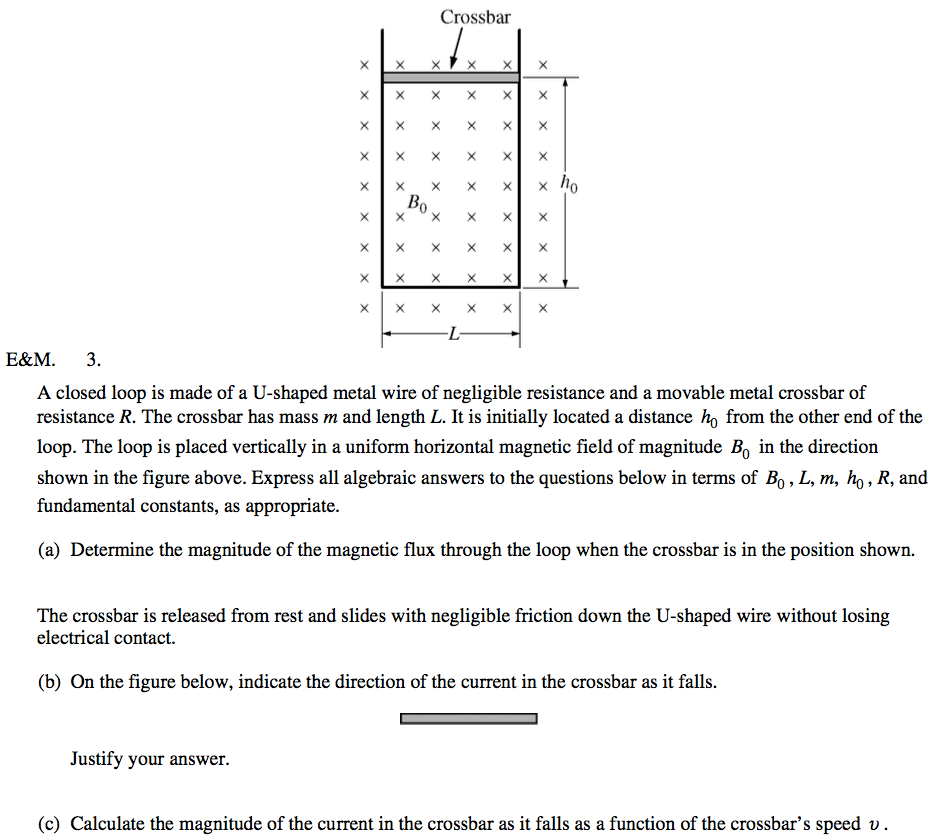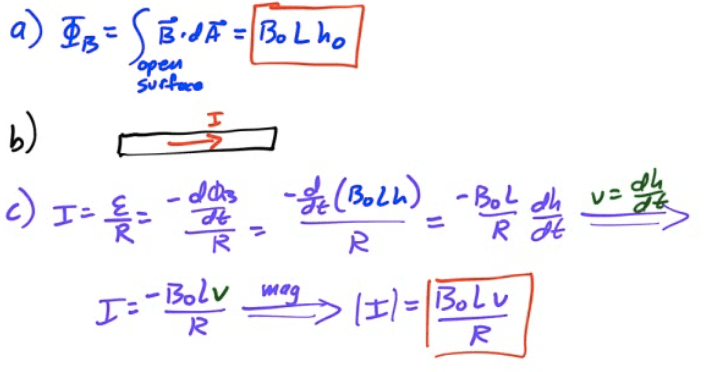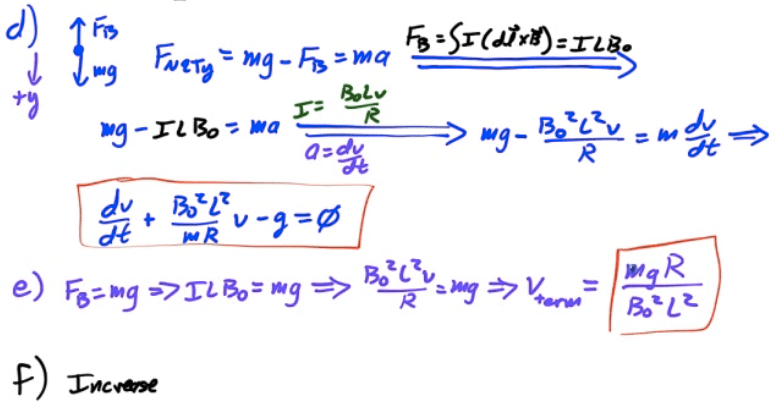# 2010 Free Response Question 3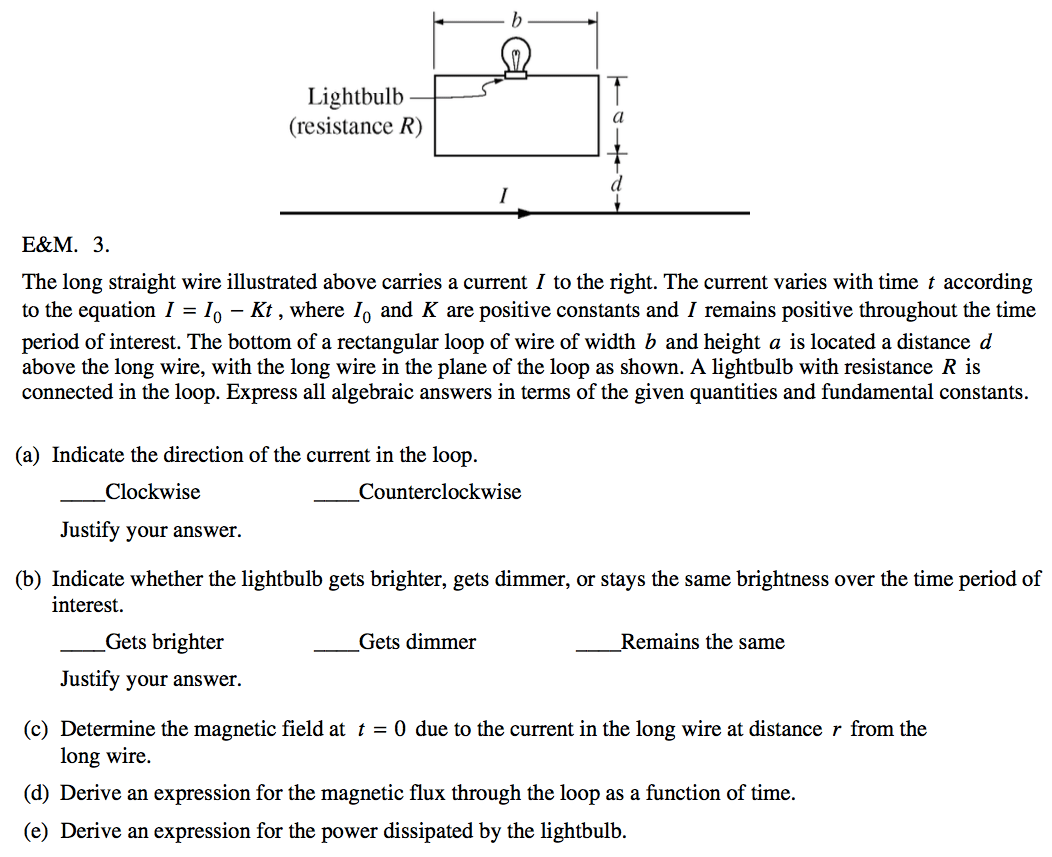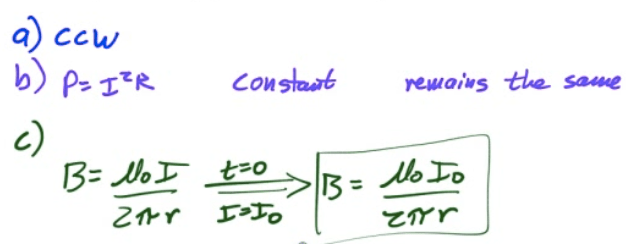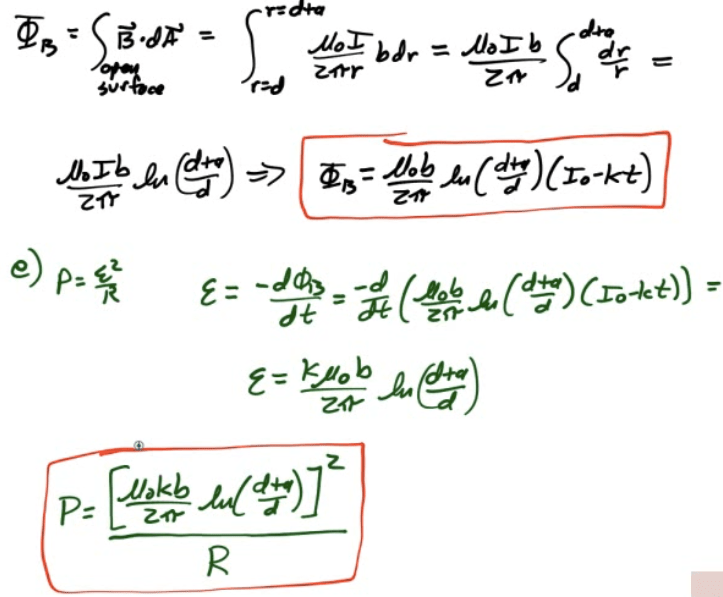# 2009 Free Response Question 3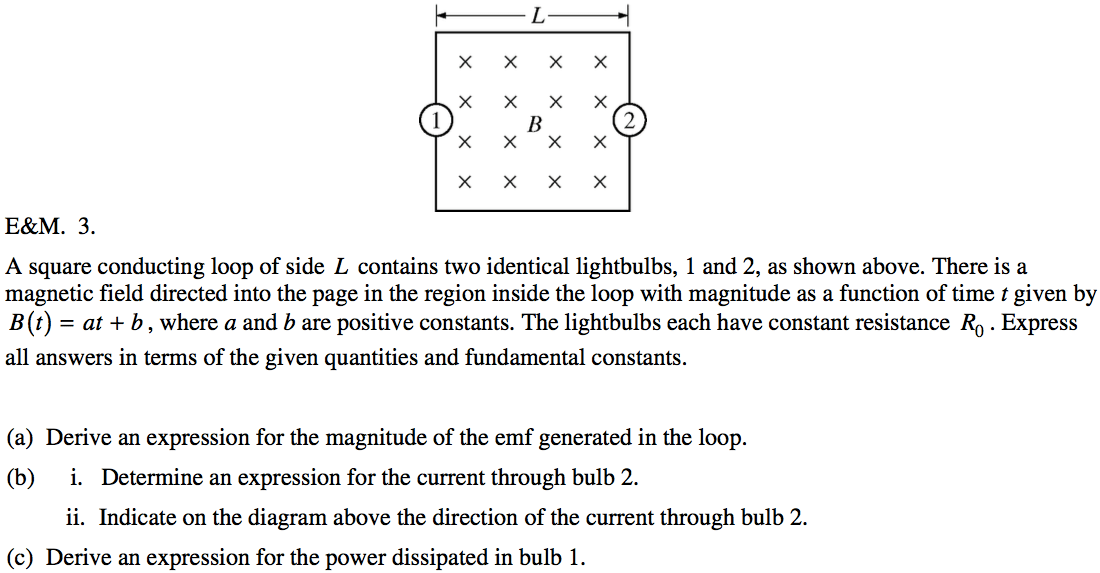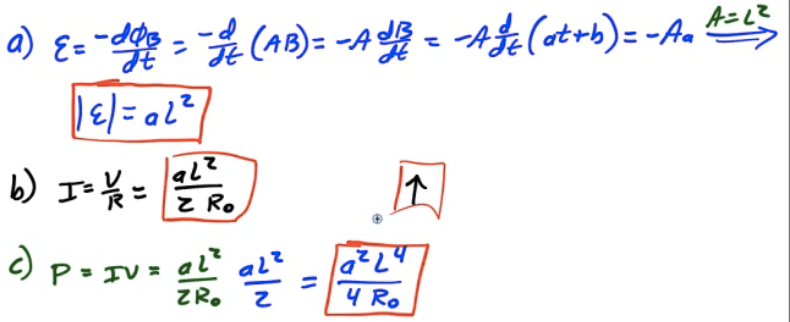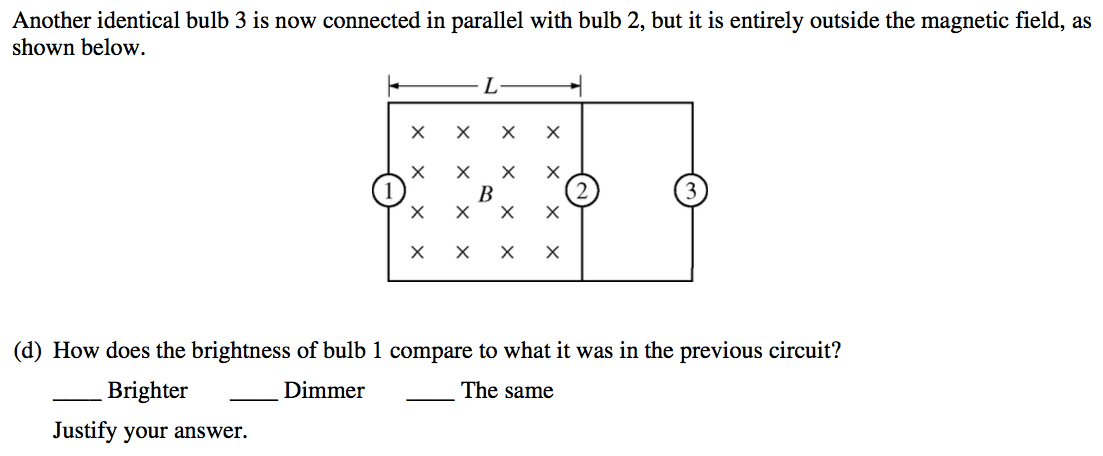# 2008 Free Response Question 3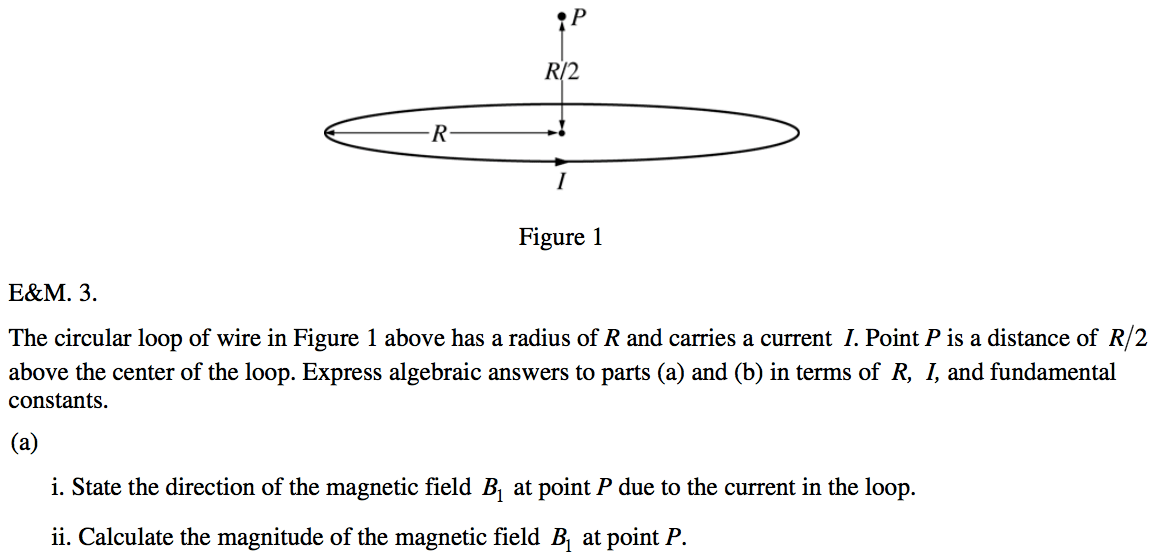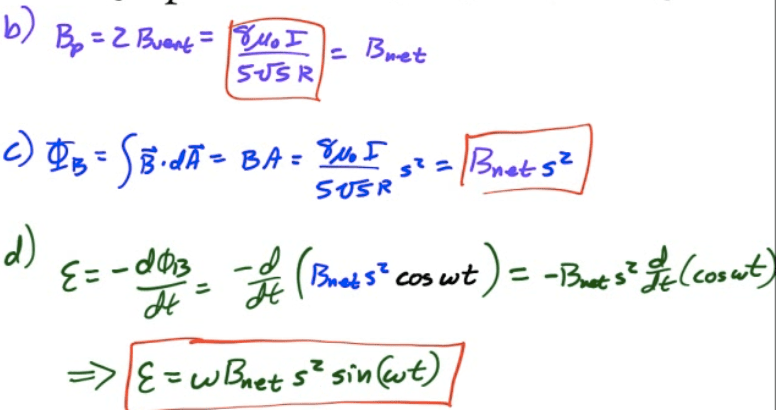# 2007 Free Response Question 3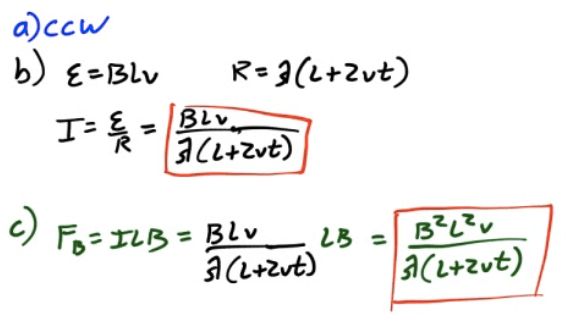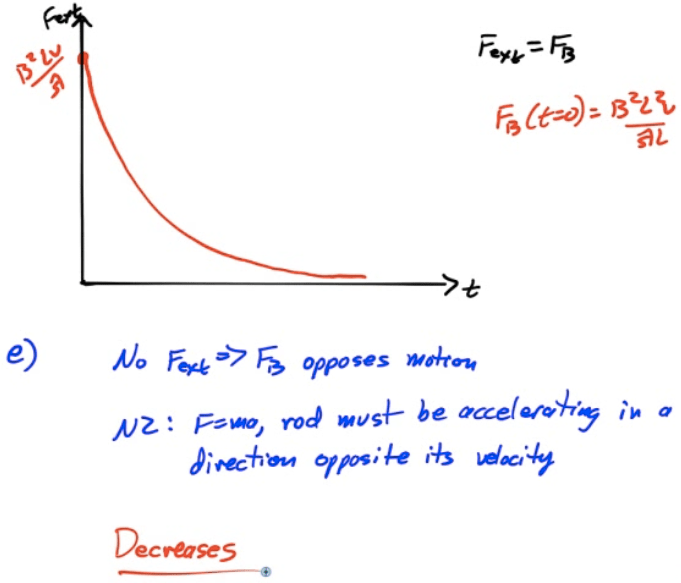# 2006 Free Response Question 3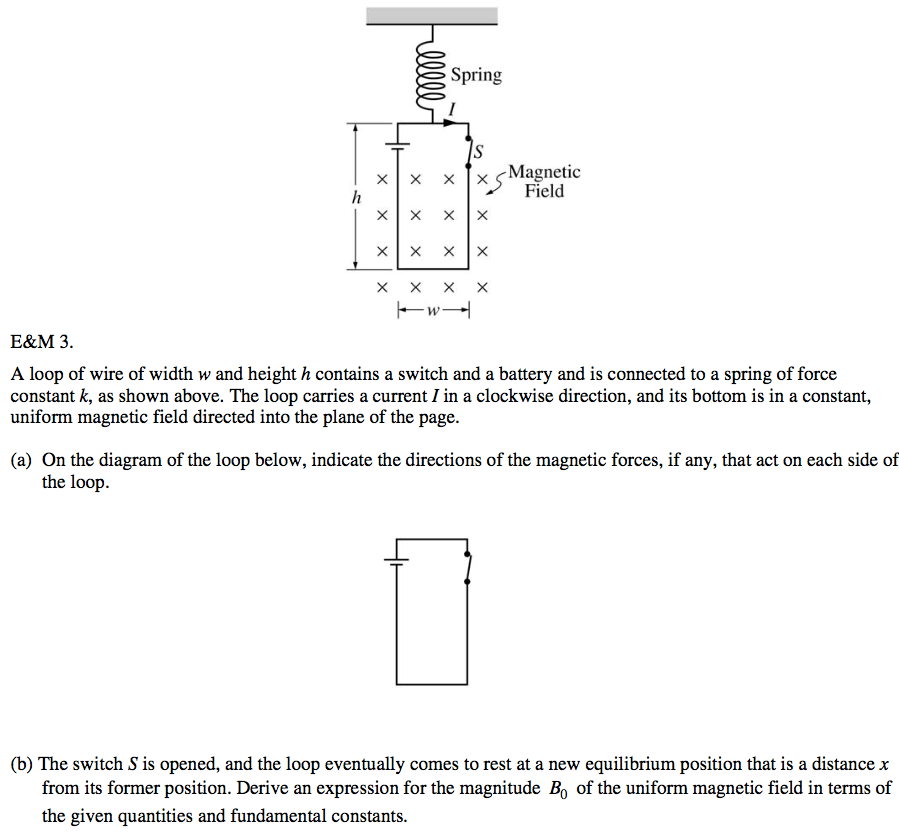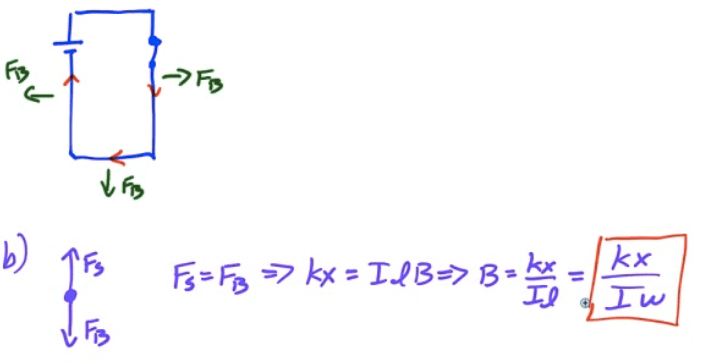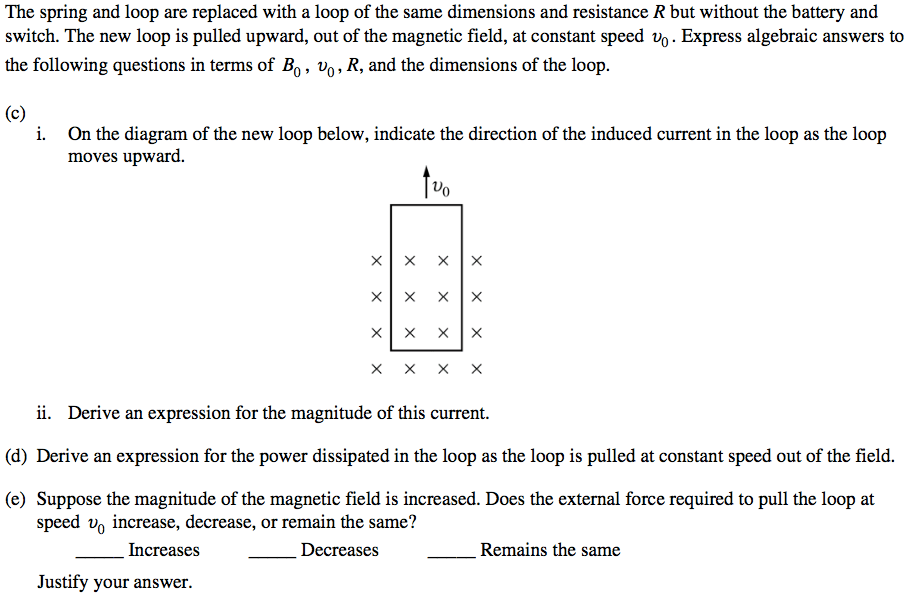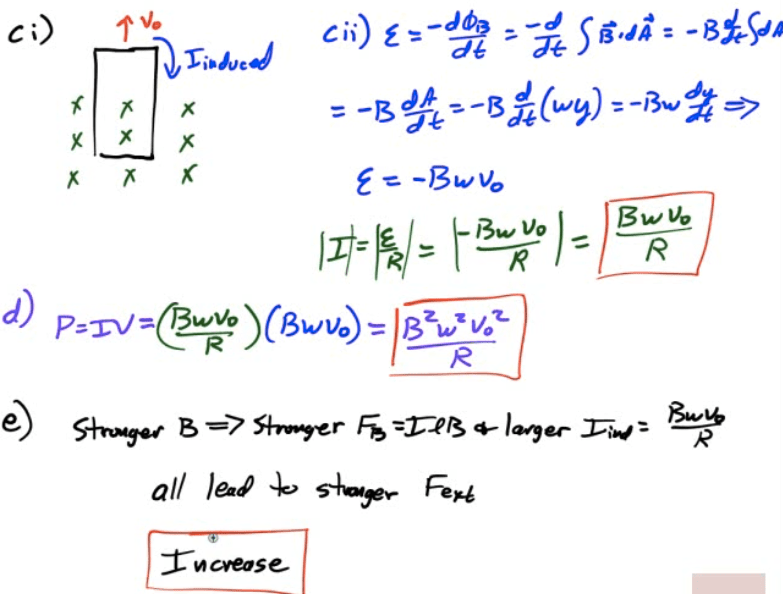# 2013 Free Response Question 3